## 13.4.4 Hamiltonian Mechanics

The Lagrangian formulation of mechanics is the most convenient for determining a state transition equation for a collection of bodies. Once the kinetic and potential energies are determined, the remaining efforts are straightforward computation of derivatives and algebraic manipulation. Hamiltonian mechanics provides an alternative formulation that is closely related to the Lagrangian. Instead of expressing second-order differential constraints on an-dimensional C-space, it expresses first-order constraints on a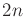-dimensional phase space. This idea should be familiar from Section 13.2. The new phase space considered here is an example of a symplectic manifold, which has many important properties, such as being orientable and having an even number of dimensions . The standard phase vector is defined as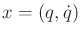; however, instead of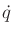,variables will be introduced and denoted as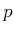. Thus, a transformation exists between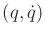and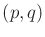. Thevariables are related to the configuration variables through a special function over the phase space called the Hamiltonian. Although the Hamiltonian formulation usually does not help in the determination of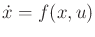, it is covered here because its generalization to optimal control problems is quite powerful. This generalization is called Pontryagin's minimum principle and is covered in Section 15.2.3. In the context of mechanics, it provides a general expression of energy conservation laws, which aids in proving many theoretical results [39,397].

The relationship betweenandcan be obtained by using the Legendre transformation [39,397]. Consider a real-valued function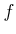of two variables,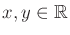. Its total differential  is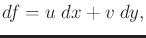(13.185)

in which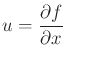and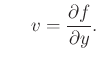(13.186)

Consider constructing a total differential that depends on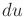and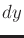, instead of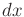and. Let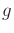be a function ofand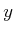defined as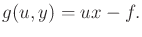(13.187)

The total differential ofis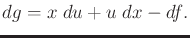(13.188)

Using (13.185) to express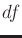, this simplifies to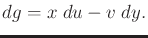(13.189)

Theand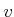variables are now interpreted as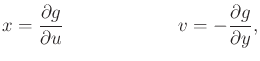(13.190)

which appear to be a kind of inversion of (13.186). This idea will be extended to vector form to arrive the Hamiltonian formulation.

Assume that the dynamics do not depend on the particular time (the extension to time-varying dynamics is not difficult; see [39,397]). Let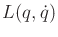be the Lagrangian function defined (13.129). Let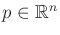represent a generalized momentum vector (or adjoint variables), which serves the same purpose asin (13.185). Each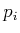is defined as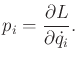(13.191)

In some literature,is instead denoted as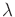because it can also be interpreted as a vector of Lagrange multipliers. The Hamiltonian function is defined as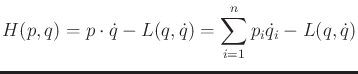(13.192)

and can be interpreted as the total energy of a conservative system . This is a vector-based extension of (13.187) in which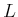and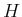replaceand, respectively. Also,and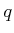are the vector versions ofand, respectively.

Considered as a function ofandonly, the total differential ofis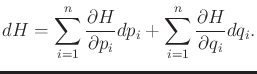(13.193)

Using (13.192),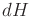can be expressed as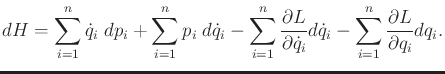(13.194)

The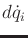terms all cancel by using (13.191), to obtain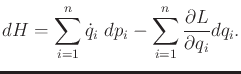(13.195)

Using (13.118),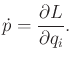(13.196)

This implies that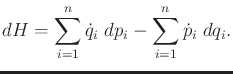(13.197)

Equating (13.197) and (13.193) yieldsequations called Hamilton's equations: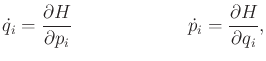(13.198)

for eachfromto. These equations are analogous to (13.190).

Hamilton's equations are equivalent to the Euler-Lagrange equation. Extremals in both cases yield equivalent differential constraints. The difference is that the Lagrangian formulation usesand the Hamiltonian uses. The Hamiltonian results in first-order partial differential equations. It was assumed here that the dynamics are time-invariant and the motions occur in a conservative field. In this case,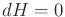, which corresponds to conservation of total energy. In the time-varying case, the additional equation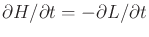appears along with Hamilton's equations. As stated previously, Hamilton's equations are primarily of interest in establishing basic results in theoretical mechanics, as opposed to determining the motions of particular systems. For example, the Hamiltonian is used to establish Louisville's theorem, which states that phase flows preserve volume, implying that a Hamiltonian system cannot be asymptotically stable . Asymptotic stability is covered in Section 15.1.1. Pontryagin's minimum principle, an extension of Hamilton's equations to optimal control theory, is covered in 15.2.3.

Steven M LaValle 2020-08-14